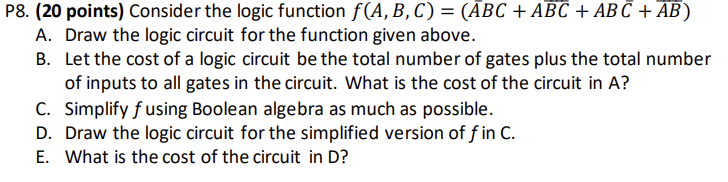Home / Expert Answers / Electrical Engineering / p8-20-points-consider-the-logic-function-f-a-b-c-overline-a-b-c-a-overline-b-c-a-b-pa868

# (Solved): P8. (20 points) Consider the logic function $$f(A, B, C)=(\overline{A B C}+A \overline{B C}+A B \ ...P8. (20 points) Consider the logic function \( f(A, B, C)=(\overline{A B C}+A \overline{B C}+A B \bar{C}+\overline{A B})$$ A. Draw the logic circuit for the function given above. B. Let the cost of a logic circuit be the total number of gates plus the total number of inputs to all gates in the circuit. What is the cost of the circuit in A? C. Simplify $$f$$ using Boolean algebra as much as possible. D. Draw the logic circuit for the simplified version of $$f$$ in $$\mathrm{C}$$. E. What is the cost of the circuit in D?

We have an Answer from Expert